# Indexing and Selecting Data with Pandas

Indexing in Pandas :
Indexing in pandas means simply selecting particular rows and columns of data from a DataFrame. Indexing could mean selecting all the rows and some of the columns, some of the rows and all of the columns, or some of each of the rows and columns. Indexing can also be known as Subset Selection.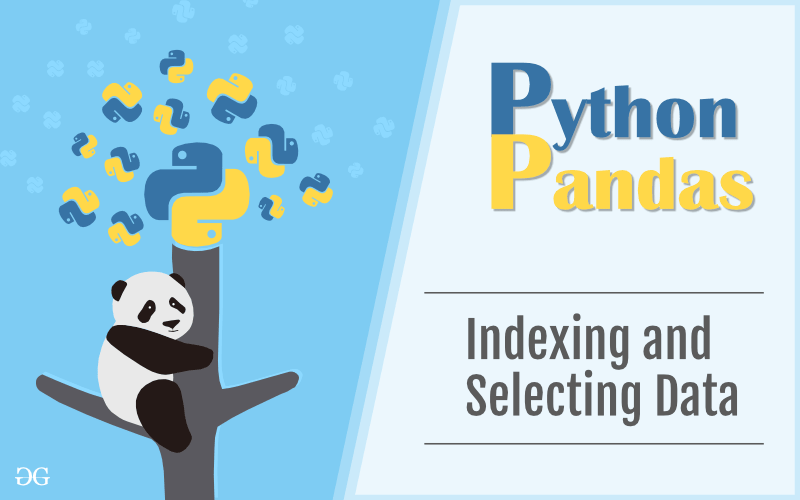Let’s see some example of indexing in Pandas. In this article, we are using “`nba.csv`” file to download the CSV, click here.

#### Selecting some rows and some columns

Let’s take a DataFrame with some fake data, now we perform indexing on this DataFrame. In this, we are selecting some rows and some columns from a DataFrame. Dataframe with dataset.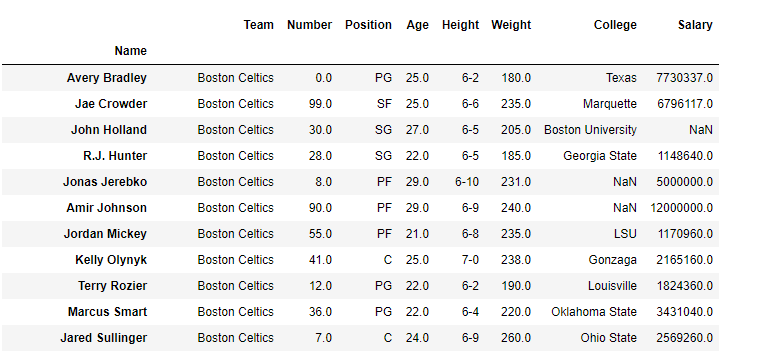Suppose we want to select columns `Age`, `College` and `Salary` for only rows with a labels `Amir Johnson` and `Terry Rozier`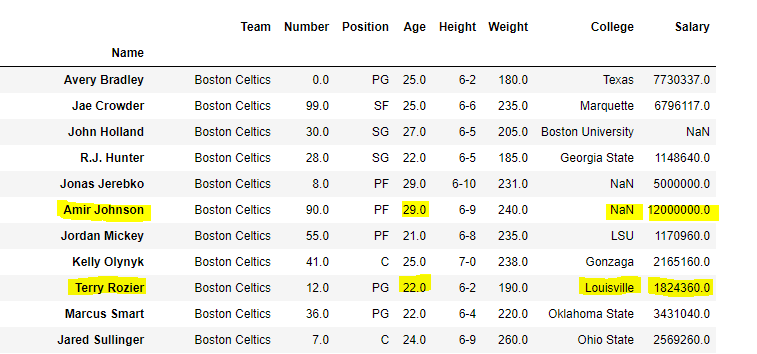Our final DataFrame would look like this: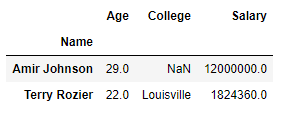#### Selecting some rows and all columns

Let’s say we want to select row `Amir Jhonson`, `Terry Rozier` and `John Holland` with all columns in a dataframe.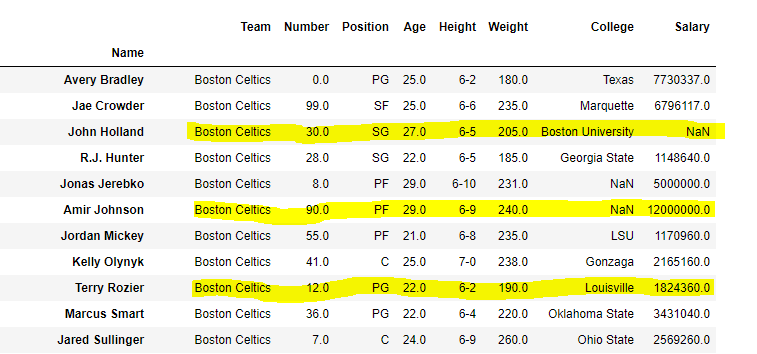Our final DataFrame would look like this: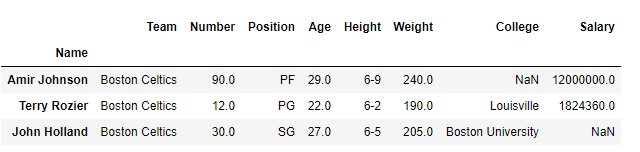#### Selecting some columns and all rows

Let’s say we want to select columns Age, Height and Salary with all rows in a dataframe.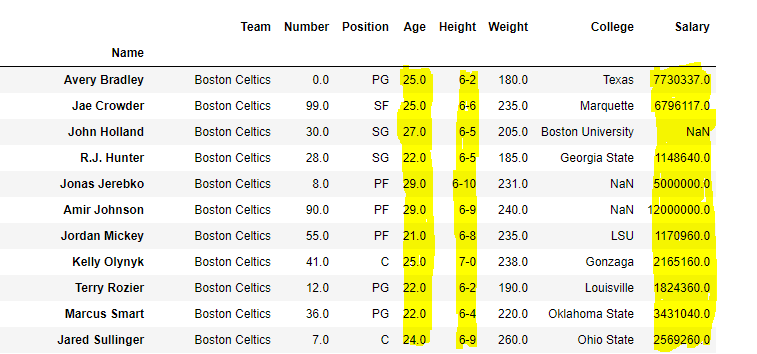Our final DataFrame would look like this: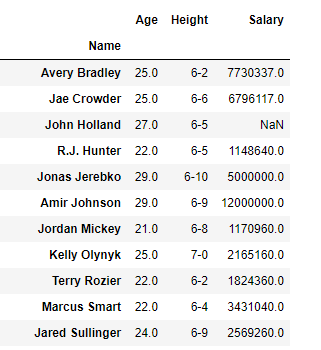### Pandas Indexing using `[ ]`, ` .loc[]`, `.iloc[ ]`, `.ix[ ]`

There are a lot of ways to pull the elements, rows, and columns from a DataFrame. There are some indexing method in Pandas which help in getting an element from a DataFrame. These indexing methods appear very similar but behave very differently. Pandas support four types of Multi-axes indexing they are:

• Dataframe.[ ] ; This function also known as indexing operator
• Dataframe.loc[ ] : This function is used for labels.
• Dataframe.iloc[ ] : This function is used for positions or integer based
• Dataframe.ix[] : This function is used for both label and integer based

Collectively, they are called the indexers. These are by far the most common ways to index data. These are four function which help in getting the elements, rows, and columns from a DataFrame.

Indexing a Dataframe using indexing operator `[]` :
Indexing operator is used to refer to the square brackets following an object. The `.loc` and `.iloc` indexers also use the indexing operator to make selections. In this indexing operator to refer to df[].

#### Selecting a single columns

In order to select a single column, we simply put the name of the column in-between the brackets

 `# importing pandas package ` `import` `pandas as pd ` ` `  `# making data frame from csv file ` `data ``=` `pd.read_csv(``"nba.csv"``, index_col ``=``"Name"``) ` ` `  `# retrieving columns by indexing operator ` `first ``=` `data[``"Age"``] ` ` `  ` `  ` `  `print``(first) `

Output: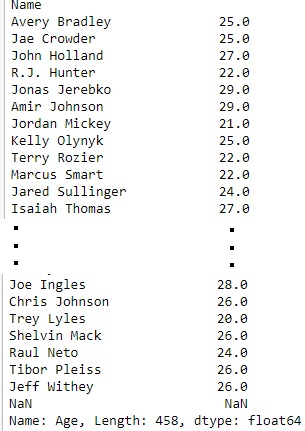#### Selecting multiple columns

In order to select multiple columns, we have to pass a list of columns in an indexing operator.

 `# importing pandas package ` `import` `pandas as pd ` ` `  `# making data frame from csv file ` `data ``=` `pd.read_csv(``"nba.csv"``, index_col ``=``"Name"``) ` ` `  `# retrieving multiple columns by indexing operator ` `first ``=` `data[[``"Age"``, ``"College"``, ``"Salary"``]] ` ` `  ` `  ` `  `first `

Output: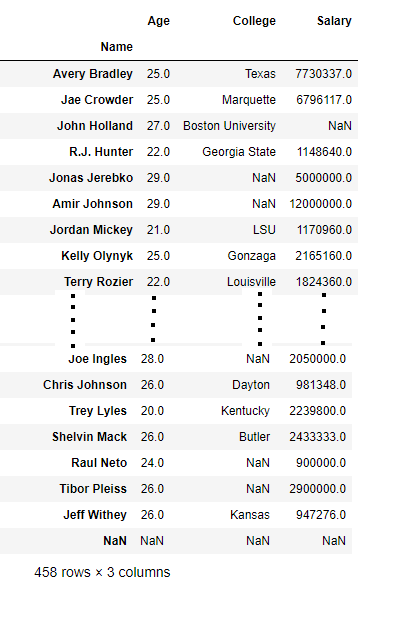Indexing a DataFrame using `.loc[ ]` :
This function selects data by the label of the rows and columns. The `df.loc` indexer selects data in a different way than just the indexing operator. It can select subsets of rows or columns. It can also simultaneously select subsets of rows and columns.

#### Selecting a single row

In order to select a single row using `.loc[]`, we put a single row label in a `.loc` function.

 `# importing pandas package ` `import` `pandas as pd ` ` `  `# making data frame from csv file ` `data ``=` `pd.read_csv(``"nba.csv"``, index_col ``=``"Name"``) ` ` `  `# retrieving row by loc method ` `first ``=` `data.loc[``"Avery Bradley"``] ` `second ``=` `data.loc[``"R.J. Hunter"``] ` ` `  ` `  `print``(first, ``"\n\n\n"``, second) `

Output:
As shown in the output image, two series were returned since there was only one parameter both of the times.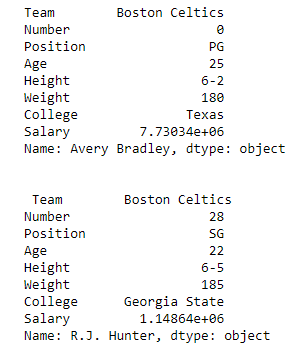#### Selecting multiple rows

In order to select multiple rows, we put all the row labels in a list and pass that to `.loc` function.

 `import` `pandas as pd ` ` `  `# making data frame from csv file ` `data ``=` `pd.read_csv(``"nba.csv"``, index_col ``=``"Name"``) ` ` `  `# retrieving multiple rows by loc method ` `first ``=` `data.loc[[``"Avery Bradley"``, ``"R.J. Hunter"``]] ` ` `  ` `  ` `  `print``(first) `

Output: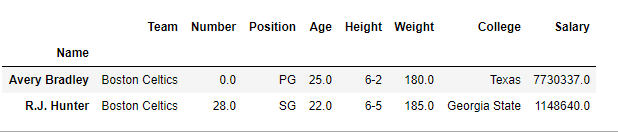#### Selecting two rows and three columns

In order to select two rows and three columns, we select a two rows which we want to select and three columns and put it in a separate list like this:

```Dataframe.loc[["row1", "row2"], ["column1", "column2", "column3"]]
```

 `import` `pandas as pd ` ` `  `# making data frame from csv file ` `data ``=` `pd.read_csv(``"nba.csv"``, index_col ``=``"Name"``) ` ` `  `# retrieving two rows and three columns by loc method ` `first ``=` `data.loc[[``"Avery Bradley"``, ``"R.J. Hunter"``], ` `                   ``[``"Team"``, ``"Number"``, ``"Position"``]] ` ` `  ` `  ` `  `print``(first) `

Output:#### Selecting all of the rows and some columns

In order to select all of the rows and some columns, we use single colon [:] to select all of rows and list of some columns which we want to select like this:

```Dataframe.loc[[:, ["column1", "column2", "column3"]]
```

 `import` `pandas as pd ` ` `  `# making data frame from csv file ` `data ``=` `pd.read_csv(``"nba.csv"``, index_col ``=``"Name"``) ` ` `  `# retrieving all rows and some columns by loc method ` `first ``=` `data.loc[:, [``"Team"``, ``"Number"``, ``"Position"``]] ` ` `  ` `  ` `  `print``(first) `

Output: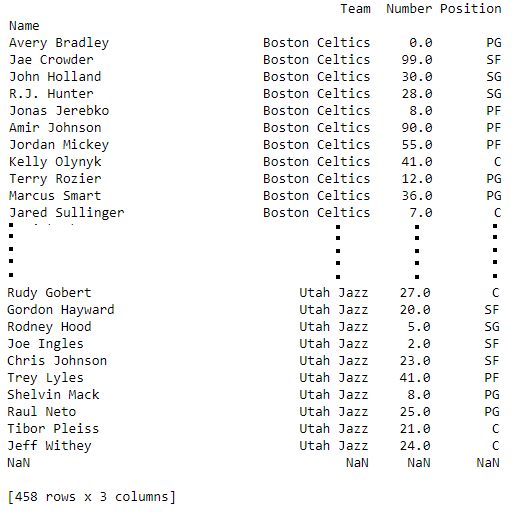Indexing a DataFrame using `.iloc[ ]` :
This function allows us to retrieve rows and columns by position. In order to do that, we’ll need to specify the positions of the rows that we want, and the positions of the columns that we want as well. The `df.iloc `indexer is very similar to `df.loc `but only uses integer locations to make its selections.

#### Selecting a single row

In order to select a single row using `.iloc[]`, we can pass a single integer to `.iloc[]` function.

 `import` `pandas as pd ` ` `  `# making data frame from csv file ` `data ``=` `pd.read_csv(``"nba.csv"``, index_col ``=``"Name"``) ` ` `  ` `  `# retrieving rows by iloc method  ` `row2 ``=` `data.iloc[``3``]  ` ` `  ` `  ` `  `print``(row2) `

Output: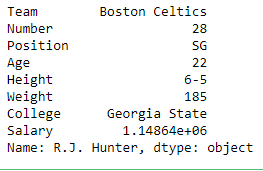#### Selecting multiple rows

In order to select multiple rows, we can pass a list of integer to `.iloc[]` function.

 `import` `pandas as pd ` ` `  `# making data frame from csv file ` `data ``=` `pd.read_csv(``"nba.csv"``, index_col ``=``"Name"``) ` ` `  ` `  `# retrieving multiple rows by iloc method  ` `row2 ``=` `data.iloc [[``3``, ``5``, ``7``]] ` ` `  ` `  ` `  `row2 `

Output: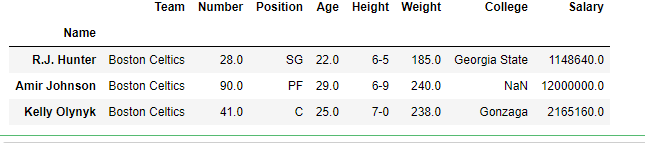#### Selecting two rows and two columns

In order to select two rows and two columns, we create a list of 2 integer for rows and list of 2 integer for columns then pass to a `.iloc[]` function.

 `import` `pandas as pd ` ` `  `# making data frame from csv file ` `data ``=` `pd.read_csv(``"nba.csv"``, index_col ``=``"Name"``) ` ` `  ` `  `# retrieving two rows and two columns by iloc method  ` `row2 ``=` `data.iloc [[``3``, ``4``], [``1``, ``2``]] ` ` `  ` `  ` `  `print``(row2) `

Output: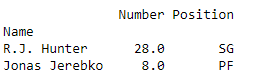#### Selecting all the rows and a some columns

In order to select all rows and some columns, we use single colon [:] to select all of rows and for columns we make a list of integer then pass to a `.iloc[]` function.

 `import` `pandas as pd ` ` `  `# making data frame from csv file ` `data ``=` `pd.read_csv(``"nba.csv"``, index_col ``=``"Name"``) ` ` `  ` `  `# retrieving all rows and some columns by iloc method  ` `row2 ``=` `data.iloc [:, [``1``, ``2``]] ` ` `  ` `  ` `  `print``(row2) `

Output: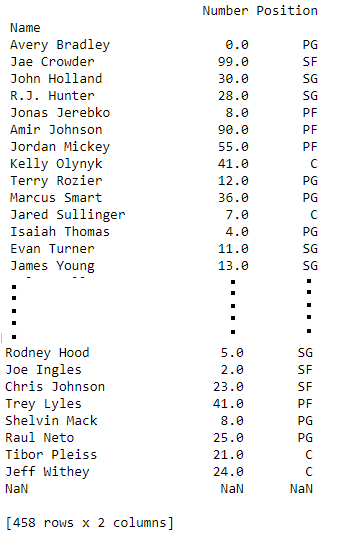Indexing a using Dataframe.ix[ ] :
Early in the development of pandas, there existed another indexer, `ix`. This indexer was capable of selecting both by label and by integer location. While it was versatile, it caused lots of confusion because it’s not explicit. Sometimes integers can also be labels for rows or columns. Thus there were instances where it was ambiguous. Generally, `ix` is label based and acts just as the .loc indexer. However, `.ix` also supports integer type selections (as in .iloc) where passed an integer. This only works where the index of the DataFrame is not integer based `.ix` will accept any of the inputs of `.loc` and `.iloc`.
Note: The .ix indexer has been deprecated in recent versions of Pandas.

#### Selecting a single row using `.ix[]` as `.loc[]`

In order to select a single row, we put a single row label in a `.ix` function. This function act similar as .loc[] if we pass a row label as a argument of a function.

 `# importing pandas package ` `import` `pandas as pd ` `  `  `# making data frame from csv file ` `data ``=` `pd.read_csv(``"nba.csv"``, index_col ``=``"Name"``) ` `  `  `# retrieving row by ix method ` `first ``=` `data.ix[``"Avery Bradley"``] ` ` `  `  `  `  `  `print``(first) ` ` `

Output: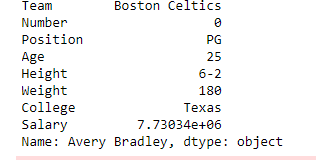#### Selecting a single row using `.ix[]` as `.iloc[]`

In order to select a single row, we can pass a single integer to `.ix[]` function. This function similar as a iloc[] function if we pass an integer in a `.ix[]` function.

 `# importing pandas package ` `import` `pandas as pd ` `  `  `# making data frame from csv file ` `data ``=` `pd.read_csv(``"nba.csv"``, index_col ``=``"Name"``) ` `  `  `# retrieving row by ix method ` `first ``=` `data.ix[``1``] ` ` `  `  `  `  `  `print``(first) `

Output: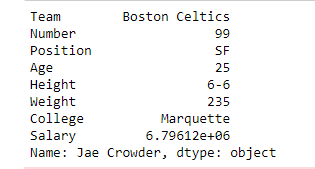#### Methods for indexing in DataFrame

Function Description
Dataframe.head() Return top` n` rows of a data frame.
Dataframe.tail() Return bottom `n` rows of a data frame.
Dataframe.at[] Access a single value for a row/column label pair.
Dataframe.iat[] Access a single value for a row/column pair by integer position.
Dataframe.tail() Purely integer-location based indexing for selection by position.
DataFrame.lookup() Label-based “fancy indexing” function for DataFrame.
DataFrame.pop() Return item and drop from frame.
DataFrame.xs() Returns a cross-section (row(s) or column(s)) from the DataFrame.
DataFrame.get() Get item from object for given key (DataFrame column, Panel slice, etc.).
DataFrame.isin() Return boolean DataFrame showing whether each element in the DataFrame is contained in values.
DataFrame.where() Return an object of same shape as self and whose corresponding entries are from self where cond is True and otherwise are from other.
DataFrame.mask() Return an object of same shape as self and whose corresponding entries are from self where cond is False and otherwise are from other.
DataFrame.query() Query the columns of a frame with a boolean expression.
DataFrame.insert() Insert column into DataFrame at specified location.

My Personal Notes arrow_drop_up
Article Tags :

6

Please write to us at contribute@geeksforgeeks.org to report any issue with the above content.